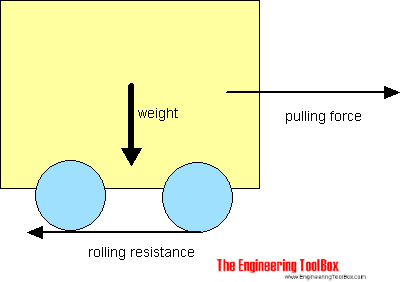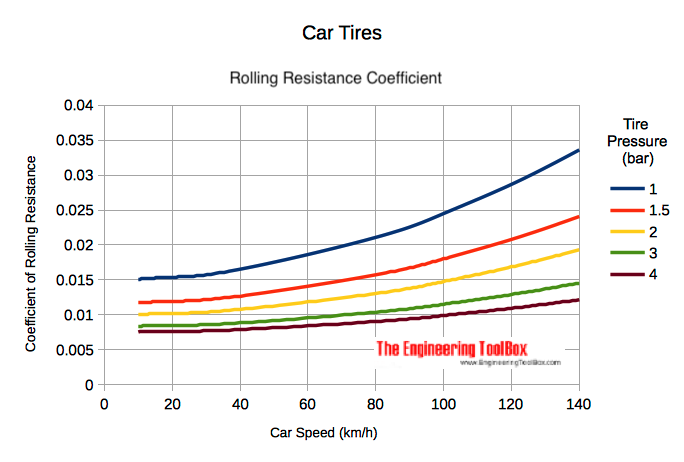Engineering ToolBox - Resources, Tools and Basic Information for Engineering and Design of Technical Applications!

# Rolling Resistance

## Rolling friction and rolling resistance.

The force that resists the motion of a body rolling on a surface is called the rolling resistance or the rolling friction.The rolling resistance can be expressed by the generic equation

Fr = c W                                   (1)

where

Fr = rolling resistance or rolling friction (N, lbf)

c = rolling resistance coefficient - dimensionless (coefficient of rolling friction - CRF)

W = m ag

= normal force - or weight - of the body (N, lbf)

m = mass of body (kg, lb)

ag = acceleration of gravity (9.81 m/s2, 32.174 ft/s2)

Note that the rolling resistance coefficient - c - is influenced by different variables like wheel design, rolling surface, wheel dimensions and more.

The rolling resistance can alternatively be expressed as

Fr = cl W / r                                (2)

where

cl = rolling resistance coefficient - dimension length (coefficient of rolling friction) (mm, in)

r = radius of wheel (mm, in)

### Rolling Friction Coefficients

Some typical rolling coefficients:

Rolling Resistance Coefficient
ccl (mm)
0.001 - 0.002 0.5 railroad steel wheels on steel rails
0.001 bicycle tire on wooden track
0.002 - 0.005 low resistance tubeless tires
0.002 bicycle tire on concrete
0.004 bicycle tire on asphalt road
0.005 dirty tram rails
0.006 - 0.01 truck tire on asphalt
0.008 bicycle tire on rough paved road
0.01 - 0.015 ordinary car tires on concrete, new asphalt, cobbles small new
0.02 car tires on tar or asphalt
0.02 car tires on gravel - rolled new
0.03 car tires on cobbles  - large worn
0.04 - 0.08 car tire on solid sand, gravel loose worn, soil medium hard
0.2 - 0.4 car tire on loose sand

### Rolling Coefficients Cars

The rolling coefficients for air filled tires on dry roads can be estimated

c = 0.005 + (1 / p) (0.01 + 0.0095 (v / 100)2)                     (3)

where

c = rolling coefficient

p = tire pressure (bar)

v = velocity (km/h)

#### Example - Wheel Pressure & Rolling Resistance Coefficient

The standard wheel pressure in a Tesla Model 3 is 2.9 bar (42 psi). The rolling friction coefficient at 90 km/h (56 mph) can be calculated from (3) as

c = 0.005 + (1 / (2.9 bar)) (0.01 + 0.0095 ((90 km/h) / 100)2)

= 0.011

Increasing the pressure to 3.5 bar reduces the rolling resitance coefficient to

c = 0.005 + (1 / (3.5 bar)) (0.01 + 0.0095 ((90 km/h) / 100)2)

= 0.010

- or

((0.011 - 0.10) / 0.011) 100% = 9%

###• 1 bar = 105 Pa = 14.5 psi
• 1 km/h = 0.6214 mph

### Example - The Rolling Resistance of a Car on Asphalt

The rolling resistance for all four wheels in a car with total weight 1500 kg on asphalt with rolling friction coefficient 0.03 can be estimated with the generic equation 1 as

Fr = 0.03 (1500 kg) (9.81 m/s2)

= 441 N

= 0.44 kN

The rolling resistance for one wheel can be calculated as

Fr = 0.03 (1500 kg / 4) (9.81 m/s2)

= 110 N

= 0.11 kN

## Related Topics

• Dynamics - Motion - velocity and acceleration, forces and torque.
• Mechanics - Forces, acceleration, displacement, vectors, motion, momentum, energy of objects and more.

## Engineering ToolBox - SketchUp Extension - Online 3D modeling!

Add standard and customized parametric components - like flange beams, lumbers, piping, stairs and more - to your Sketchup model with the Engineering ToolBox - SketchUp Extension - enabled for use with the amazing, fun and free SketchUp Make and SketchUp Pro .Add the Engineering ToolBox extension to your SketchUp from the SketchUp Pro Sketchup Extension Warehouse!

Translate

## Privacy

We don't collect information from our users. Only emails and answers are saved in our archive. Cookies are only used in the browser to improve user experience.

Some of our calculators and applications let you save application data to your local computer. These applications will - due to browser restrictions - send data between your browser and our server. We don't save this data.

## Citation

• Engineering ToolBox, (2008). Rolling Resistance. [online] Available at: https://www.engineeringtoolbox.com/rolling-friction-resistance-d_1303.html [Accessed Day Mo. Year].

Modify access date.

. .

#### Scientific Online Calculator6 24